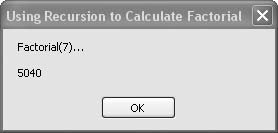# Recipe 6.23. Using Recursion to Calculate Factorials

#### Problem

You want to study a sample of Visual Basic's ability to define recursive functions, or you need a factorial function for smaller integers.

#### Solution

Sample code folder: Chapter 06\Factorial

Create a Factorial() function that recursively calls itself.

#### Discussion

The code in this recipe does not represent the most efficient way to calculate factorials for larger integers. You'll want to use a standard For…Next loop or similar process when working with larger numbers, simply because each recursive function call uses up stack space and adds a little overhead. However, recursive functions can be quite useful in some programming situations. A simple recursive function that calculates the factorial of a number is a great way to understand recursion.

The factorial of a number N is the product of all numbers from 1 to N. For example, the factorial of 3 is calculated as 3 x 2 x 1, which results in a value of 6. The Factorial() function returns the value 1 if it is passed a value of zero; otherwise, it returns the passed value times the factorial of the next smaller integer. Study the Select Case lines of code in the function to see how this is accomplished:

` Public Function Factorial(ByVal number As Decimal) As Decimal     Select Case number         Case Is < 0             Throw New Exception("Factorial: Bad argument")         Case Is = 0             Return 1         Case Else             Return number * Factorial(number - 1)     End Select End Function `

Calling the Factorial() function from inside its own code is what recursion is all about. All pending returns are literally stacked up until the value of the passed number finally reaches zero, at which time the pending multiplications all happen in a hurry. As a result of the way this recursion works, if you request the Factorial() of a large number, you run the risk of running out of stack memory or of numeric over-flow. With Decimal variables, as shown in the previous code, the largest value you can pass to the function without overflow is just 27. Of course, the factorial of 27 is a huge number, and the answer is exact when using Decimal values. You might consider switching the algorithm to use Double values to find approximations of even larger factorials.

The following lines demonstrate the Factorial() function by calculating and displaying the factorial of 7:

` Dim result As New System.Text.StringBuilder Dim number As Decimal = 7 result.AppendLine("Factorial(" & number & ")… ") result.AppendLine() result.Append(Factorial(number)) MsgBox(result.ToString()) `

Figure 6-23 shows the results of calculating the factorial of 7.

##### Figure 6-23. Calculating the factorial of a number with the Factorial( ) functionVisual Basic 2005 Cookbook: Solutions for VB 2005 Programmers (Cookbooks (OReilly))
ISBN: 0596101775
EAN: 2147483647
Year: 2006
Pages: 400

Similar book on Amazon